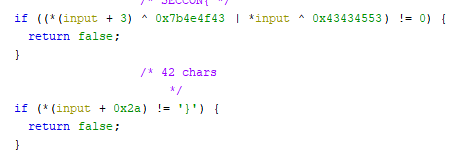# SECCON 2022 - eguite

We are give an ELF and EXE file (the same challenge - you can pick your enemy) with the simple task to crack it license.

Not sure if it's on purpose (or due to using sandbox/VM) but running it fails so we can't get any more information from running it.

We can load it into Ghidra and start our analysis. By checking the `main`, we can learn that it uses `eframe`. Any by searching for it, we can find out that's a Rust's package for GUI (desktop) applications.

We can find the interesting function going from `Successfully validated!` string to xref and then into the `click` handler.

First the function checks if our input starts with `SECCON{` and if it's 42 characters long. If we pass that we can proceed with more thorough validation.SECCON{ has to be at the beginning and we need 42 characters...

The next part is a bit fuzzy how it looks, but we can see the following section a couple of times

so we can assume that some sections in the flag are connected with the char `-` between them. It will be clear after the next part.

And next, our input is split into parts with the following calls

In the above excerpt, we start from character 7 taking 12 characters and we after that it's being parsed as a number in base-16. We store it in `first_num` variable.

We do similar starting from character 20 and taking 6 -> `second_num`, starting from 27 and taking 6 -> `third_num` and fopr the last group from 34th character spanning for 8 characters -> `fourth_num`.

Having those values we need to make sure that they fulfill the following relation between them

We can employ `z3` here to find us the correct numbers:

``````from z3 import *
s = Solver()
first_num = BitVec('first_num', 64)
second_num = BitVec('second_num', 64)
third_num = BitVec('third_num', 64)
fourth_num = BitVec('fourth_num', 64)

s.add(third_num ^ second_num ^ fourth_num == 0xf9686f4d)
s.check()
print(s.model())
``````

and they will be quickly obtained as

``````[second_num = 5929746,
fourth_num = 4190049136,
third_num = 9242415,
first_num = 152980487201880]
``````

Converting them to hex, and putting `-` between them gives us the flag `SECCON{8b228b98e458-5a7b12-8d072f-f9bf1370}`.